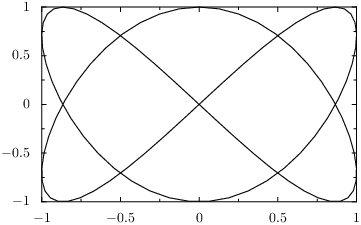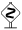# PyX — Example: graphs/lissajous.py

0.2 KB
43.3 KB
49.1 KB
24.7 KB
20.5 KB

## Plotting a parametric function```from math import pi
from pyx import *

g = graph.graphxy(width=8)
g.plot(graph.data.paramfunction("k", 0, 2*pi, "x, y = sin(2*k), cos(3*k)"))
g.writeEPSfile("lissajous")
g.writePDFfile("lissajous")
g.writeSVGfile("lissajous")
```

### Description

This example shows how to use a `paramfunction` as a data source for a graph. `paramfunction` defines a parameter variable name given as a string in the first argument, a minimal and a maximal value given as the second and third argument and an expression string as forth argument. This expression string assigns a tuple of expressions to a tuple of data names. As usual the styles will decide what those data names are responsible for.Like for the function, you can also access external data and functions in your expression. Suppose we want to provide the data for this example by means of the following python function:

```def lissajousdata(k):
return sin(2*k), cos(3*k)
```

Then we would need to modify the plot command to:

```g.plot(graph.data.paramfunction("t", min, max, "x, y = f(t)",
context={"f": lissajousdata}))
```

Note, that `t` and `f` in the string expression stand for `k` and `lissajousdata` respectively. You can also use the same names in the expression if you like.When you try this, you will notice, that you need to add `sin` and `cos` to the list of imported symbols from the math module. While this should be expected, it is more interesting to note, that it was not necessary to import those functions before. The reason is, that the string expressions are evaluated in a certain context. This context does not only contain the symbols given in the `context` keyword argument, but in addition some standard mathematical functions (and constants) including `sin` and `cos`. See the reference manual for a complete list.

For the common case of plotting a parametric function providing data for `x` and `y` by means of an external function, there is a special `paramfunctionxy` which directly calls the external function instead of using a context in a string expression. A `lambda` expression could serve to provide the data inline, which results in:

```g.plot(graph.data.paramfunctionxy(lambda k: (sin(2*k), cos(3*k)), 0, 2*pi))
```

Note that the external expression is provided in the first argument here. Since the system just need to call the function, the parameter variable name does not have to be provided to `paramfunctionxy`.